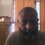# Problem in Calculus related to the minimalization of a definite integral

I could not post this note elsewhere, hence am posting it here

This relates to the problem involving minimization of the integral (between 0 to pi/2) of the expression (sin(x) - (px^2+qx_)^2

Conceptualizing the problem geometrically, the above could be translated as =minimizing the distance between the sine curve and the parabola y=px^2 +qx

My feeling is this can happen if and only if the zero crossings and the peaks of the curves match, ie if px^2+qx at x=pi = 0 or if px^2+qx has its maximum at pi/2

Both these result in the following expression between p and q q = -p*pi

One idea seems to be to simplify the expression by expressing it as a difference between the integrals of sin(x) and (px^2+qx) separately (which can be interpreted as the difference between areas under curves) which would also minimize the squared difference , since their peaks match (possible links to orthogonality etc)

The other idea seems to be let the maximum of the parabola be equal to 1 at pi/2

One could possibly get more sophisticated by requiring tangency between the curves at pi/2 but in any case, the four numbers seem to be of equal magnitude but opposite in sign which seems to give zero

One could of course also look at taylors expansion of sin(x)

The above seems to be related to least squares of a sin curve by a parabola or vice-versa in a least squares approximation

It would have made more sense to look at polynomials involving sin(x) and ,more generally sin(nx) which is related to the fourier series expansion / fourier transform of px^2+qxNote by Sundar R
6 years, 10 months ago

This discussion board is a place to discuss our Daily Challenges and the math and science related to those challenges. Explanations are more than just a solution — they should explain the steps and thinking strategies that you used to obtain the solution. Comments should further the discussion of math and science.

When posting on Brilliant:

• Use the emojis to react to an explanation, whether you're congratulating a job well done , or just really confused .
• Ask specific questions about the challenge or the steps in somebody's explanation. Well-posed questions can add a lot to the discussion, but posting "I don't understand!" doesn't help anyone.
• Try to contribute something new to the discussion, whether it is an extension, generalization or other idea related to the challenge.
• Stay on topic — we're all here to learn more about math and science, not to hear about your favorite get-rich-quick scheme or current world events.

MarkdownAppears as
*italics* or _italics_ italics
**bold** or __bold__ bold
- bulleted- list
• bulleted
• list
1. numbered2. list
1. numbered
2. list
Note: you must add a full line of space before and after lists for them to show up correctly
paragraph 1paragraph 2

paragraph 1

paragraph 2

[example link](https://brilliant.org)example link
> This is a quote
This is a quote
    # I indented these lines
# 4 spaces, and now they show
# up as a code block.

print "hello world"
# I indented these lines
# 4 spaces, and now they show
# up as a code block.

print "hello world"
MathAppears as
Remember to wrap math in $$ ... $$ or $ ... $ to ensure proper formatting.
2 \times 3 $2 \times 3$
2^{34} $2^{34}$
a_{i-1} $a_{i-1}$
\frac{2}{3} $\frac{2}{3}$
\sqrt{2} $\sqrt{2}$
\sum_{i=1}^3 $\sum_{i=1}^3$
\sin \theta $\sin \theta$
\boxed{123} $\boxed{123}$

## Comments

There are no comments in this discussion.

×

Problem Loading...

Note Loading...

Set Loading...